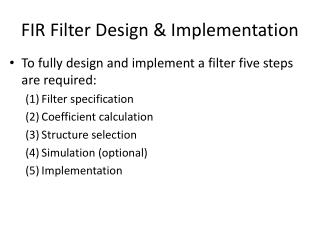Download PresentationFIR Filter Design & Implementation

# FIR Filter Design & Implementation - PowerPoint PPT Presentation

FIR Filter Design & Implementation. To fully design and implement a filter five steps are required: (1) Filter specification (2) Coefficient calculation (3) Structure selection (4) Simulation (optional) (5) Implementation. Software Tools for Designing Filter.I am the owner, or an agent authorized to act on behalf of the owner, of the copyrighted work described.
Download Presentation## FIR Filter Design & Implementation

Download Policy: Content on the Website is provided to you AS IS for your information and personal use and may not be sold / licensed / shared on other websites without getting consent from its author.While downloading, if for some reason you are not able to download a presentation, the publisher may have deleted the file from their server.

- - - - - - - - - - - - - - - - - - - - - - - - - - E N D - - - - - - - - - - - - - - - - - - - - - - - - - -
Presentation Transcript
1. FIR Filter Design & Implementation • To fully design and implement a filter five steps are required: (1) Filter specification (2) Coefficient calculation (3) Structure selection (4) Simulation (optional) (5) Implementation

2. Software Tools for Designing Filter • MATLAB provides some advanced tools for filters design • Signal Processing Tool (SPTool) • Filter Design (FDATool) • There are also some free software packages to design filters • Our main problem is how to implement the filter given the coefficients • So we will design FIR filters using the relatively simple method of Windowing -- write Matlab scripts

3. Lowpass Filter Specification

4. Window Method : Step 1 • First stage of this method is to calculate the coefficients of the ideal filter. • This is calculated as follows:

5. Window Method : Step 2 • Second stage of this method is to select a window function based on the passband or attenuation specifications, then determine the filter length based on the required width of the transition band

6. Window Method : Step 2 • The normalized transition bandwidth as listed in the table is defined as • Example: If we choose the Hamming window, and specify a transition band from 6kHz to 7kHz, with sampling frequency at 40kHz, then the filter order is calculated as

7. Window Method : Step 3 • The third stage is to calculate the set of truncated or windowed impulse response coefficients, h[n]: for

8. Using Matlab's fir1() • Instead of doing the design start from the ideal reponse, we shall use Matlab's fir1() function • We will design a FIR filter with the following specification • sampling frequency = 8000 • passband edge frequency = 1200 • stopband edge frequency = 1800 • minimum stopband attenuation = 40 dB • By using the Hamming window, the stopband attenuation specification is met • By default, fir1() uses the Hamming window • For more information, please refer to the Matlab on-line help

9. Using Matlab's fir1() fsamp = 8000; fp = 1200; % passband fs = 1800; % stopband fc = (fp+fs)/2; % cutoff % default using Hamming window, compute order N = round(3.3*fsamp/(fs-fp)); str=sprintf('Filter order = %d', N); disp(str); wc = 2*fc/fsamp; % normalize to pi radian b=fir1(N,wc); freqz(b,1,512,fsamp); filtdesign.m

10. FIR Filter Structure - Direct Form

11. Linear Phase FIR • For linear phase FIR, such as those designed with the window method, the coefficients have to be symmetrical • We can then make use of the symmetry to reduce the multiplications

12. Example of Symmetrical FIR - Even Length

13. Example of Symmetrical FIR - Odd Length

14. FIR Filters Design with FDATool • The Filter Design and Analysis Tool (FDATool) is a graphical user interface (GUI) for designing, quantizing, and analyzing digital filters. • It includes a number of advanced filter design techniques and supports all the filter design methods in the Signal Processing Toolbox. • designing filters by setting filter specifications; • analyzing designed filters; • converting filters to different structures; and • quantizing and analyzing quantized filters. • Open the FDATool by typing "fdatool" at theMATLAB command window.